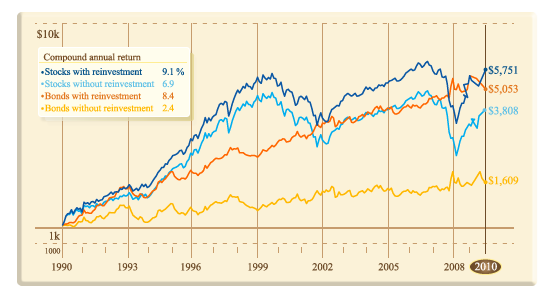# Dividend cash flowYou want to value stock dividends that are projected to grow forever at a constant rate $g$, using a discount rate of $r$. If the dividend in the first period is $D$, what is the value of this cash flow?

Assume that $r > g$.

×